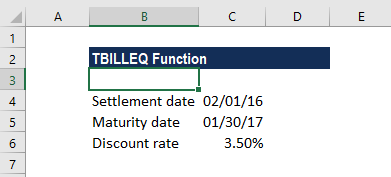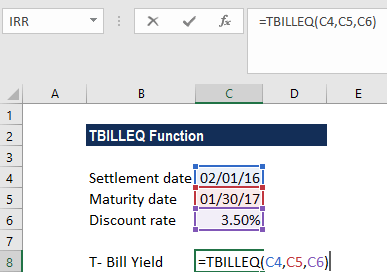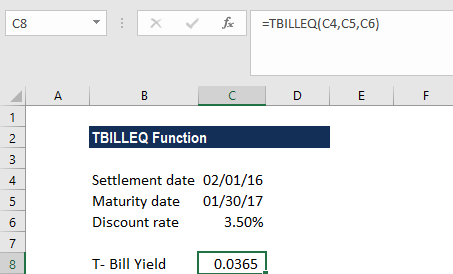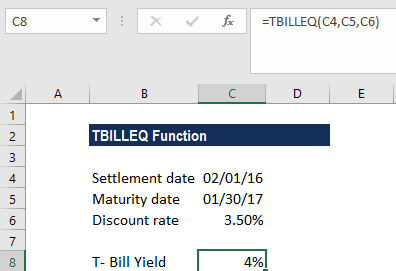# TBILLEQ Function

Calculates the bond-equivalent yield of a T-bill

## What is the TBILLEQ Function?

The TBILLEQ Function is categorized under Excel Financial functions. The function helps calculate the bond-equivalent yield of a T-bill.

In financial analysis, if we wish to compare T-bills yields with bond yields, we can use TBILLEQ.

### Formula

=TBILLEQ(settlement, maturity, discount)

The TBILLEQ function uses the following arguments:

1. Settlement (required argument) – This is the settlement date of the T-bill. It is the date after the issue date when the T-bill is traded to the buyer.
2. Maturity (required argument) – The date when the T-bill will expire. It must be greater than and within one year of the settlement date. This is so, as the life of T-bills is less than one year.
3. Discount (required argument) – The T-bill discount rate. We can take from the risk-free investment rate if unavailable.

While entering the dates in the argument, they should be:

• References to cells containing dates; or
• Dates returned from formulas.

### How to use the TBILLEQ Function in Excel?

To understand the uses of the TBILLEQ function, let’s consider an example:

#### Example

We wish to calculate the bond-equivalent yield for a Treasury bill with a settlement date of February 1, 2016, maturity date of January 30, 2017, and a discount of 3.5%.The formula to use is:TBILLEQ is calculated as TBILLEQ = (365 x rate)/(360–(rate x DSM)), where DSM is the number of days between settlement and maturity computed according to the 360 days per year basis.

We get the result below:We change the format to a percentage basis:### Things to remember about the TBILLEQ Function

1. The TBILLEQ function was introduced in Excel 2003 and is available in all subsequent Excel versions.
2. The settlement and maturity arguments are truncated to integers.
3. #NUM! error – Occurs when:
• Settlement date is ≥ maturity date.
• Maturity date is more than 1 year after the settlement date.
• Discount argument is less than or equal to 0.
4. #VALUE! error – Occurs when:
• Settlement date is an invalid date.
• Maturity date is an invalid date.
• The arguments provided are non-numeric.

Thanks for reading CFI’s guide to important Excel functions! By taking the time to learn and master these functions, you’ll significantly speed up your financial analysis. To learn more, check out these additional CFI resources:

• Excel Functions for Finance